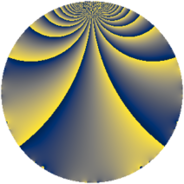# Properties

 Label 546.2.bbLevel $546$ Weight $2$ Character orbit 546.bb Rep. character $\chi_{546}(269,\cdot)$ Character field $\Q(\zeta_{6})$ Dimension $76$ Newform subspaces $1$ Sturm bound $224$ Trace bound $0$

# Related objects

## Defining parameters

 Level: $$N$$ $$=$$ $$546 = 2 \cdot 3 \cdot 7 \cdot 13$$ Weight: $$k$$ $$=$$ $$2$$ Character orbit: $$[\chi]$$ $$=$$ 546.bb (of order $$6$$ and degree $$2$$) Character conductor: $$\operatorname{cond}(\chi)$$ $$=$$ $$273$$ Character field: $$\Q(\zeta_{6})$$ Newform subspaces: $$1$$ Sturm bound: $$224$$ Trace bound: $$0$$

## Dimensions

The following table gives the dimensions of various subspaces of $$M_{2}(546, [\chi])$$.

Total New Old
Modular forms 240 76 164
Cusp forms 208 76 132
Eisenstein series 32 0 32

## Trace form

 $$76q - 76q^{4} + 10q^{7} + 4q^{9} + O(q^{10})$$ $$76q - 76q^{4} + 10q^{7} + 4q^{9} - 2q^{13} + 12q^{15} + 76q^{16} + 18q^{19} - 8q^{21} - 42q^{25} - 10q^{28} - 10q^{30} + 12q^{31} + 72q^{33} - 4q^{36} + 12q^{37} - 42q^{39} + 2q^{42} - 2q^{43} + 8q^{46} - 10q^{49} + 10q^{51} + 2q^{52} - 24q^{55} + 8q^{57} - 8q^{58} - 12q^{60} + 78q^{61} + 2q^{63} - 76q^{64} - 24q^{66} - 48q^{67} + 30q^{69} - 54q^{73} - 18q^{76} - 12q^{78} - 60q^{79} - 4q^{81} - 24q^{82} + 8q^{84} + 8q^{85} - 8q^{91} - 32q^{93} - 24q^{94} + 66q^{97} + O(q^{100})$$

## Decomposition of $$S_{2}^{\mathrm{new}}(546, [\chi])$$ into newform subspaces

Label Dim. $$A$$ Field CM Traces $q$-expansion
$$a_2$$ $$a_3$$ $$a_5$$ $$a_7$$
546.2.bb.a $$76$$ $$4.360$$ None $$0$$ $$0$$ $$0$$ $$10$$

## Decomposition of $$S_{2}^{\mathrm{old}}(546, [\chi])$$ into lower level spaces

$$S_{2}^{\mathrm{old}}(546, [\chi]) \cong$$ $$S_{2}^{\mathrm{new}}(273, [\chi])$$$$^{\oplus 2}$$